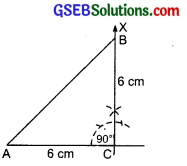# GSEB Solutions Class 7 Maths Chapter 10 Practical Geometry Ex 10.5

Gujarat Board GSEB Textbook Solutions Class 7 Maths Chapter 10 Practical Geometry Ex 10.5 Textbook Questions and Answers.

## Gujarat Board Textbook Solutions Class 7 Maths Chapter 10 Practical Geometry Ex 10.5Question 1.
Construct the right-angled ∆PQR, where m∠Q = 90°, QR = 8 cm and PR =10 cm.
Solution:
Steps of construction:
I. Draw a line segment QR = 8 cm.
II. At Q, construct ∠RQX = 90°.
III. With centre at R and radius 10 cm, draw an arc to cut $$\overrightarrow{\mathrm{QX}}$$ at P.
IV. Join PR.Thus, ∆PQR is the required right-angled triangle.

Question 2.
Construct a right-angled triangle whose hypotenuse is 6 cm long and one of the legs is 4 cm long.
Solution:
Steps of construction:
I. Draw a line segment MN = 4 cm.
II. Construct ∠NMX = 90° at M
III. With centre at N and radius 6 cm, draw an arc to cut $$\overrightarrow{\mathrm{MX}}$$ at L.
IV. Join LN.
Thus, the ∆LMN is the required right-triangled triangle.Question 3.
Construct an isosceles right-angled triangle ABC, where m∠ACB = 90° and AC = 6 cm.
Solution:
Steps of construction:
I. Draw a line segment AC = 6 cm.
II. At C, construct ∠ACX = 90°.
III. With centre C and radius AC = 6 cm, draw an arc to cut $$\overrightarrow{\mathrm{CX}}$$ at B.
IV. Join BA.
Thus, ∆ABC is the required isosceles right-angled triangle.